﻿ Deformation and Breakup of an Axisymmetric Falling Drop under Constant Body Force

### Deformation and Breakup of an Axisymmetric Falling Drop under Constant Body Force

A. Bakhshi, D.D. Ganji, M. GorjiOPEN ACCESSPEER-REVIEWED

## Deformation and Breakup of an Axisymmetric Falling Drop under Constant Body Force

A. Bakhshi1,, D.D. Ganji1, M. Gorji1

1Department of Mechanical Engineering, Babol University of science and technology, Babol, Iran

### Abstract

The secondary breakup of liquid drops, accelerated by a constant body force, is examined for small density differences between the drops and the surrounding fluid. a density ratio of ten has been studied. We used Volume of Fluid (VOF) method to simulate the breakup. The breakup is controlled by the Eötvös number (Eo), the Ohnesorge number (Oh), and the viscosity and density ratios. If viscous effects are small (small Oh), the Eotvos number is the main controlling parameter. At a density ratio of ten, as Eo increases the drops break up in a backward facing bag, transient breakup, and a shear breakup mode. Similar breakup modes have been seen experimentally for much larger density ratios. Although a backward facing bag is seen at low Oh, where viscous effects are small, comparisons with simulations of inviscid flows show that the bag breakup is a viscous phenomenon, due to boundary layer separation and the formation of a wake. At higher Oh, where viscous effects modify the evolution, the simulations show that the main effect of increasing Oh is to move the boundary between the different breakup modes to higher Eo. The results are summarized by “breakup maps” where the different breakup modes are shown in the Eo–Oh plane for different values of the viscosity and the density ratios.

### At a glance: Figures

12
Prev Next

• Bakhshi, A., D.D. Ganji, and M. Gorji. "Deformation and Breakup of an Axisymmetric Falling Drop under Constant Body Force." International Journal of Partial Differential Equations and Applications 3.1 (2015): 1-6.
• Bakhshi, A. , Ganji, D. , & Gorji, M. (2015). Deformation and Breakup of an Axisymmetric Falling Drop under Constant Body Force. International Journal of Partial Differential Equations and Applications, 3(1), 1-6.
• Bakhshi, A., D.D. Ganji, and M. Gorji. "Deformation and Breakup of an Axisymmetric Falling Drop under Constant Body Force." International Journal of Partial Differential Equations and Applications 3, no. 1 (2015): 1-6.

 Import into BibTeX Import into EndNote Import into RefMan Import into RefWorks

### 1. Introduction

The deformation and breakup of liquid droplets is encounteredin a wide range of industrial applications as well as in natural situations. Engineering examples are found in diesel engines andother types of combustion applications, electro-sprayed paint andcosmetics, ink-jet printers, turbines, micro-fluidics, cooling systems, etc. In the case of geophysical phenomena, examples abound,ranging from volcanic eruption and tephra formation to rain phenomenon. A thorough description of fragmentation phenomenafor a variety of applications can be found in Villermaux . Interfacial interaction could change depending on the circumstances a droplet is dispersed in. For example, conditions in a shock-tube would be different than a drop tower. The main difference between the two mentioned situations is the approach of velocity change. In contrast to step velocity change in shock tube tests, droplet accelerates more gradually in drop towers due to gravity. Here we investigate the behavior of a single droplet experiencing gravitational force. It will be demonstrated that different sorts of deformation take place including flattening, breakup, coalescence, torus formation, liquid bridges creation and breakup, etc. Thus, the breakup mechanism of single falling drops is not only helpful for direct applications such as rain drop investigations, but also assists to clarify the behavior of deformable mono-dispersed phases with different geometries in complex flows.

To understand the droplet dynamics, many experimental, theoretical, and numerical studies have been performed. Pilch and Erdman  described the breakup modes separately for a single drop experiencing an external flow under different ranges of the Weber number. Basically, droplet breakup can be categorized mainly according to Weber and Eötvös numbers for aero-break and free-fall respectively, in six modes: vibrational mode, bag mode, bag-stamen mode, stripping mode, wave crest stripping mode and catastrophic breakup. O’Brian  and Burger et al.  (1983), studied the free-fall of oil and gallium drops, respectively and presented the different modes of breakup for liquid–liquid two-phase systems. According to their results, it could be understood that the classification described by Pilch and Erdman  is also valid for liquid–liquid systems. Besides the work of Pilch and Erdman , several experimental investigations are performed to classify the breakup regimes. Krzeczkowski  made a study of deformation and fragmentation of liquid droplets due to an external air stream. Using image processing, Krzeczkowski  introduced four main breakup modes in terms of Weber number consisting of bag, bag-jet, transition, and shear modes. He also studied the breakup duration and the dependency of this parameter on the viscosity ratio. He found that the viscosity ratio does not affect the mechanism and breakup period notably and the most dominant factor is the Weber number. Chou et al. , Chou and Faeth , and Dai and Faeth  carried out a series of experimental studies on temporal properties of shear, bag, and multimode breakup regimes. Several factors such as deformation, droplet velocities, breakup time, critical Weber number and drag coefficient are systematically presented using the shadowgraph method. The multimode regime in the work of Daiand Faeth , is divided into two main regimes called bag-plume and plume-shear modes. Cao et al. , using high speed camera and shadowgraphs, identified a new mode close to multimode regime called dual-bag breakup for a liquid droplet in a uniform air jet flow. The mechanism of this mode consists of two bag fragmentations of the main drop and the core drop generated in the first breakup. It was postulated that the dual-breakup is different from the observation of Krzeczkowski  and Dai and Faeth  for the similar Weber range obtained in shock tubes. It was argued that the dual-breakup mode is due to dissimilar kinds of disturbances made in uniform jet flow. Cao et al.  mentioned that bag or bag-stamen modes can be seen for the lower limit of dual-bag regime, as well as shear or explosive regimes for higher limit. Recently Guildenbecher et al.  reviewed the technical literature for experimental methods and morphology of droplet atomization for Newtonian and non-Newtonian fluids. They highlighted that ‘‘the mechanism leading to fragmentation of the bag is not well understood’’ while the study of this mode is particularly imperative because it ascertains the criteria for secondary atomization. They also report a map table as a function of Weber number for different breakup modes and presented a relatively comprehensive description on what we know about the mechanism of each mode.

Recognizing various breakup modes for droplet fragmentation is the basic step for analyzing the mechanism of breakup. However, what is seen in the experiments for highly unstable regions demonstrate that modes are combined and multi-mode-fragmentation happens. It means that in most cases, shear, bag, wave crest, and other modes are included in the entire procedure of the fragmentation. This occurs because of different local conditions related to local velocity, fragment size, etc.

The overlapping of breakup modes occurs for a wide range of Weber numbers. This issue is especially remarkable for the range of 20 <We< 80, where different types of breakup are reported. It should be noted that the studies mentioned here used shock tube or wind tunnel experiments. In contrast to drop deformation and breakup in wind tunnel or shock tubes, smaller number of studies can be found investigating the falling droplet fragmentation. Reyssat et al.  experimentally explored an unstable range of water drops falling in air. They demonstrated an identifiable, specific class of bag breakup for drops with diameter much larger than capillary length. The distorted shape of such drops consists of a thin aqueous sheet surrounded by air and bounded at the bottom by a torus. During the falling process, as the instability intensifies, the sheet ruptures and a deformed toroidal rim comes into sight. This high-speed fragmentation is followed by destabilization and breakup of liquid bridges due to Savart–Rayleigh–Plateau instability (Rayleigh, ). In the end, a very large drop falling in air decays in several stable fragments of individual sizes maller than the capillary length. Reyssat et al.  extended their work for very large water drops, larger than 3 cm, and showed that multiple bags are formed because of Rayleigh–Taylor-like instabilities. A similar scenario including inflation, disintegration and destabilization of liquid bridges come to pass for each of the unstable fragments. Recently the secondary breakup of liquid drops, etc. has been studied by several authors [14-20].

In this letter the secondary breakup of liquid drops, accelerated by a constant body force, is examined for small density differences between the drops and the surrounding fluid. a density ratio of ten has been studied. We used Volume of Fluid (VOF) method to simulate the breakup. The breakup is controlled by the Eötvös number (Eo), the Ohnesorge number (Oh), and the viscosity and density ratios.

### 2. Numerical Method

2.1. Governing Equations

In the VOF method, the transport equation for the VOF function, α, of each phase is solved simultaneously with a single set of continuity and Navier–Stokes equations for the whole flow field. Considering the two fluids as Newtonian, incompressible, and immiscible, the governing equations can be written as: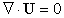(1)(2)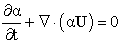(3)

Where U is the fluid velocity, p the pressure, f the gravitational force, and FS volumetric representation of the surface tension force. The bulk density ρb and viscosity μb are computed as the averages over the two phases, weighted with the VOF function α:(4)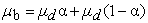(5)

Where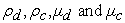are the densities and the viscosities of the two phases. In the VOF method, α is advected by the fluids. For incompressible flows, this is equivalent to a conservation law for the VOF function, and therefore ensures the conservation of mass.

The surface tension force, FS, is modeled as a volumetric force by the Continuum Surface Force (CSF) method . It is only active in the interfacial region and formulated as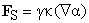where γ is the interfacial tension andis the curvature of the interface.

2.2. Interface Sharpening

In OpenFOAM, the fluid interface is sharpened by introducing the artificial compression term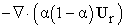into Eq. (3). Thus, the VOF equation (Eq. (3)) becomes:(6)

The artificial compression velocity Ur is given by: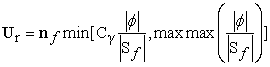(7)

Where nf is the normal vector of the cell surface, ϕ is the mass flux, Sf is the cell surface area, and Cγ is an adjustable coefficient, the value of which can be set between 0 and 4. Physically, we can interpret Ur as a relative velocity between the two fluids, which arises from the density and viscosity change across the fluid interface.

By taking the divergence of the compression velocity Ur, the conservation of the VOF function is guaranteed.  The termensures that this artifact is only active in the interfacial area where 0<α <1. The level of compression depends on the value of Cγ: there is no compression with, a moderate compression with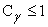, and an enhanced compression with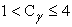[23, 24].

2.3. VOF Smoothing

In the VOF method, the fluid interface is implicitly represented by the VOF function, the value of which sharply changes over a thin region. This abrupt change of the VOF function creates errors in calculating the normal vectors and the curvature of the interface, which are used to evaluate the interfacial forces. These errors induce non-physical parasitic currents in the interfacial region . An easy way to suppress these artifacts is to compute the interface curvature κ from a smoothed VOF function, which is calculated from the VOF function α by smoothing it over a finite region around the fluid interface . Thus, the curvature of the fluid interface is: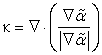(8)

Whereas in all other equations, the non-smoothed VOF function α is used.

In this study we applied the smoother proposed by Lafaurieet al. , namely a Laplacian filter that transforms the VOF function α into a smoother function: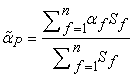(9)

Where the subscript Pdenotes the cell index and f denotes the face index. The interpolated value αf at the face center is calculated using linear interpolation. The application of this filter can be repeated times to get a smoothed field. It should be stressed that smoothing tends to level out high curvature regions and should therefore be applied only up to the level that is strictly necessary to sufficiently suppress parasitic currents.

2.4. Numerical Setup and Discretization

Our numerical simulations were performed with the finite-volume-based code OpenFOAM on co-located grids. The PISO (pressure-implicit with splitting of operators) scheme is applied for pressure–velocity coupling . The transient terms are discretized using a first order implicit Euler scheme, controlling the time step by setting the maximum Courant number to 0.3. Higher Courant numbers were found to lead to a distortion of the interface due to increased parasitic currents. We also examined the performance of a second order implicit backward time integration scheme for one of the test cases on droplet breakup described in Section 3.3. Since the difference in breakup time was less than 1%, we used first order Euler schemes throughout this work. For spatial discretization, a second order TVD scheme with van Leerlimiter was used. To ensure the boundedness of the VOF function, we used a special discretization scheme developed by OpenCFDLtd., interface Compression, with the MULES (Multidimensional Universal Limiter with Explicit Solution) explicit solver .

The flow domains were meshed with hexahedral cells using Blockmesh, an internal mesh generator of OpenFOAM. At the channel walls, no-slip and zero contact angle boundary conditions were specified. This contact angle boundary condition is used to correct the surface normal vector, and therefore adjusts the curvature of the interface in the vicinity of the wall. A uniform velocity and zero-gradient for pressure and VOF function α were applied at the inlet. At the outlet, we imposed a fixed-valued (atmospheric) pressure boundary condition and zero-gradient for velocity and VOF function α.

### 3. Non-dimensional Numbers

In the case of a falling droplet in a motionless media the acceleration due to gravity, surface tension between the fluids, viscosity of fluids, initial diameter of the droplet and density of fluids are the main parameters. Considering these parameters, different non-dimensional groups governing the behavior of the droplet are proposed by researchers. A list of important dimensionless numbers for this study is as follows:

Eotvos number: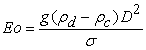Ohnesorge number (based on droplet properties):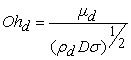Ohnesorge number (based on continuous phase properties):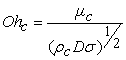Density ratio:Viscosity ratio:where, g is the gravitational acceleration, D is the initial diameter of the drop,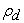and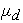are density and viscosity of the droplet,are density and viscosity of the continuous phase, respectively. And σ is surface tension coefficient.

### 4. Numerical Validation

To make sure that the surface tension effect is properly implemented in this model, two test cases are considered. First it has been checked an initial square drop in a 2D domain can freely deform to a circular drop and secondly the coalescence of two static drops that merge to become a single circular drop is simulated. In the first test, physical setup is a square drop with a length of 8 cm which is placed in the middle of the 16 cm* 16 cm computational domain. In the second test, physical setup is two circular drops with radius 1 cm which is placed in the 8 cm * 8 cm computational domain.

In all simulations in this paper we used,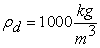,,,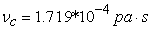and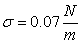.

Figure 1. Free deformation of a static droplet from a square initial shape a circular finale shape

Figure 1 and Figure 2 show the results. Both cases show deformation to the final circular drop, indicating that the surface tension effect is correctly implemented.

### 5. Results and Discussions

5.1. Effect of Eo at Small Oh

When Oh is small and surface tension is much more important than viscous stresses, Oh has little influence on the breakup and Eo is the only controlling parameter. Here, we present results for different Eo when Oh is small. When a drop is set into motion by a constant body force, the hydrodynamic pressure is higher at the poles and lower at the equator and the drop deforms into an oblate ellipsoid. This deformation is opposed by the surface tension. Depending on the relative strength of the pressure forces and the surface tension, measured by Eo, different breakup modes are observed.

Figure 3. Effect of Eoon the deformation of drops. The boundaries of the column do not indicate the actual boundaries of thecomputational domain. The gap between two successive drops in each column represents the distance the drop travels at a fixed time interval and the last interface is plotted at t = a) 1.15s, b) 0.88s, c) 0.69s, d) 0.56s, e) 0.49s, f) 0.41s , g) 0.36s

In Figure 3, the effect of Eo is presented for density ratio of 10 and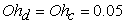. The simulations are done using a moving coordinate system where the origin is fixed at the centroid of the drop. The domain has dimensions of five and thirty times the initial drop diameter in the radial and axial directions, respectively. The centroid of the drop is fixed at aposition three times the initial drop diameter below the top boundary. The evolution of the drop is shown for seven different values of Eo. In each column, the drop interface is plotted at fixed time intervals. The separation between two successive drops is equal to the distance that the drop travels during the time interval.

In (a), the drop is shown for Eo = 9. As the drop starts falling, the back side becomes flat while the front side retains a rounded shape. Then the drop deformation gets more pronounced and the back of the drop becomes increasingly more convex and eventually the drop deforms into a thin disk-like shape that moves at a nearly steady state. The drop shown in (b) for Eo = 15 evolves in the same way until it has deformed into a disk-like shape. Then the thickness of the drop near the symmetry axis continues to decrease, and most of the drop fluid moves outward toward the edge of the drop. Finally, the center of the front surface is pushed upward, forming a backward-facing bag. At this stage, most of the drop fluid is contained in the annular-shaped rim. As time progresses, the bag expands both radially outward and vertically upward. Experimental evidence indicates that the drop will eventually break into small drops. When Eo is further increased to 39 in (c), a different mode of breakup is observed. The initial deformation is not very different from the previous cases, and an indentation develops on the back surface, but instead of deforming into a disk-like shape, the drop remains relatively thick near the symmetry axis and the edge of the drop is swept back in the downstream direction. A large wave then develops on the drop interface and as this wave propagates, the drop deforms in an erratic manner. The evolution of the drop shown in (f) for Eo = 60 reveals another mode of deformation. The initial evolution is similar to the previous cases, but the results are different at later times. As the indentation at the top progressively deepens, the drop does not deform into a thin disk-like shape. Instead, the edge of the drop is deflected in the downstream direction and the drop starts to break into small drops from its edge. By increasing Eo as we see in (e), (f) and (g) the similar behavior is observed but the intensity and speed of the breakup increases.

Based on these results, the evolution of drops with density ratio of 10 at a small Oh can be classified into four categories in order of increasing Eo: steady deformation, formation of a backward-facing bag, transient breakup with a complex shape, and stripping or shearing of a film from the edge of the drop. It is evident from Figure 3 that drops breaking up in the backward-facing mode travel a much longer distance than those breaking up in the shear breakup mode. Also note that for the same breakup mode, the rate of drop deformation increases as Eo increases.

Figure 4. Centroid velocity versus time for the drops shown in Figure 3. The results are presented for Eo = 9, 15, 39, 60, 78, 96 and 132. Ohd = Oho = 0.05

Figure 4 shows the centroid velocity of the drop Vc plotted versust for the drops shown in Figure 3 By increasing the Eo the rate of acceleration of falling droplet increases thus droplet falls with higher speed and deforms sooner. The lowest Eo drop (Eo = 9) asymptotically reaches a steady state velocity, but the other drops all slow down as they start deforming. The Eo = 15 drop also reaches a steady velocity. The drops that undergo bag breakup first behave like the Eo = 9 drop, but as the bag forms, the drops slow down rapidly. The rest of the drops all slow down rapidly as they are stretched perpendicular to the flow, and all speed up again as the edges strip.

5.2. Effect of Oh

Figure 5 - Figure 6 illustrate the effect of the Ohnesorge number the (non-dimensional viscosity) for drops with a finite density ratio of 10. The drops are shown at several times.

In the Figure 5 Ohd = Oho = 0.05, 0.125, and 0.25, from left to right, and Eo = 15. The Oh = 0.05 case (a) has already been shown in Figure 3(b), but is included here for comparison. The initial deformation of all three drops is similar, but whereas the Oh = 0.05 drop (a) deforms into a backward-facing bag, the other two drops almost reach a steady state shape. Of those, the less viscous drop (b) is flatter.

In the Figure 6 Eo is increased to 132 and the evolution of the drops is presented for the same three values of Oh as the Figure 5 In (a), the drop is already shown in Figure 3 (g) is included for reference. This drop undergoes a so-called shear (or boundary stripping) breakup. The Oh = 0.125 drop (b) shows a similar evolution as the drop in (a), although the rate of deformation is reduced slightly. The center portion of the drop still contains a significant amount of drop fluid and formation of a backward-facing bag, which requires the formation of a very thin film of fluid near the symmetry axis, does not occur.

Based on the results shown in Figure 5 and Figure 6, it is clear that increasing both Oho and Ohd simultaneously results in a translation of the boundaries between the breakup modes to higher Eo.

Figure 6. Effect of Oh on deformation of drops with Eo = 132. Time steps are fixed and end time is 0.36s

### 6. Conclusion

The deformation and breakup of axisymmetric drops, accelerated by a constant body force, have been studied by numerical simulations. Results are presented for density ratio of 10. For low Ohnesorge numbers, the Eotvos number and the density ratio are the main controlling parameters. At low density ratios the drop deforms, but does not break up for Eo less than about 10. For 10<Eo<22 (approximately), the drop breaks up by the formation of a backward facing bag. For Eo larger than about 40, the drop evolves into a forward-facing bag.

The formation of a forward-facing bag takes place very quickly (the drop has moved only 3–4 times its initial diameter when the bag is formed) and is essentially an inviscid phenomenon. The formation of a backward-facing bag, on the other hand, takes significantly longer (the drop has moved 8–10 times its initial diameter). A comparison with results obtained by an inviscid vortex method shows that the backward-facing bag is a viscous phenomenon, due to the formation of a low pressure wake behind the drop. Furthermore, the surface area of the drop increases at a faster rate in the forward-facing bag mode.

As Oh is increased, the effect of the viscosity reduces the rate of deformation. At low Eo, while the drop flattens, its center does not drain completely and a backward-facing bag does not form. As Eo becomes larger, the edges of the drop are pulled outward and sheared off, leading to a “skirted” drop.

### References

  E. Villermaux, Fragmentation. Ann. Rev. Fluid Mech. 39, 419-446, 2007.In article  M. Pilch,C.A. Erdman, Int. J. Multiphase Flow 13 (6), 741-757,1987.In article  V.O’Brian,J. Met. 18, 549-552, 1961.In article  M.Burger, W.Schwalbe, D.S. Kim, H.Unger, H.Hohmann, H. Schins,J. Heat Transfer 106 (4), 728-735, 1983.In article  M. Pilch, C.A. Erdman, Int. J. Multiphase Flow 13 (7), 851-857, 1989.In article  S. A Krzeczkowski, Int. J. Multiphase Flow 6, 227-239, 1980.In article  W.-H.Chou, L.-P.Hsiang, G.M.Faeth, Int. J. Multiphase Flow 23 (4), 651-669, 1997.In article  W.-H.Chou, G.M.Faeth, Int. J. Multiphase Flow 24 (6), 889-912, 1998.In article  Z. Dai, G. M. Faeth, Int. J. Multiphase Flow 27 (2), 217-236, 2001.In article  X.-K.Cao, Z.-G.Sun, W.-F.Li, H.-F.Liu, Z.-H.Yu, Phys. Fluids 19 (5), 057103, 2007.In article  D. R. Guildenbecher, C. López-Rivera, P. E. Sojka, Exp. Fluids 46 (3), 371-402, 2009.In article  E. Reyssat,F. Chevy, A. L. Biance, L. Petitjean, D. Quere, EPL 80, 34005, 2007.In article  L. Rayleigh, Proc. Lond. Math. Soc. 10, 4-13, 1878.In article  A. Fakhri, M. H. Rahimian, Commun. Nonlinear Sci. Numer. Simulat. 14, 3045-3046, 2009.In article  A. Vahabzadeh, M. Fakour, D.D. Ganji, IJPEDA. 2 (6), 96-104, 2014.In article  J.Q.Feng, J. Fluid Mech. 658, 438-462, 2010.In article  M. Fakour, A. Vahabzadeh, D.D. Ganji, CSTE.In article  J. Han, G. Tryggvason, Phys. Fluids 11 (12), 3650, 1999.In article  M. Fakour, D.D. Ganji, M. Abbasi, CSTE. (2014).In article  A. Vahabzadeh, M. Fakour, D.D. Ganji, I.Rahimi Petroudi, Cent. Eur. J. Eng. 4 (2014) 341-355.In article CrossRef  JU.Brackbill, D. B. Kothe, C. A. Zemach, J Comput Phys, 100, 335-354, 1992.In article  H. G. Weller, Technical report, OpenCFD Ltd, 2008.In article  E. Berberovic, N. P. van Hinsberg, S. Jakirlic´,I. V.Roisman, C. Tropea, PhysRev E, 79, 036306, 2009.In article  S. S. Deshpande, L. Anumolu, M. F. Trujillo, Comput Sci Disc, 5:014016, 2012.In article  R. Scardovelli, S. Zaleski,Annu Rev Fluid Mech, 31, 567-603, 1999.In article  B. Lafaurie, C. Nardone, R. Scardovelli, S. Zaleski, G. Zanetti, J Comput Phys, 113, 134-47, 1994.In article  Rusche H. Computational fluid dynamics of dispersed two-phase flows at high phase fractions. Ph.D. Thesis, Imperial College of Science, Technology and Medicine; 2002.In article  OpenCFD Ltd., OpenFOAMU serguide, OpenCFD Ltd., 2009.In article